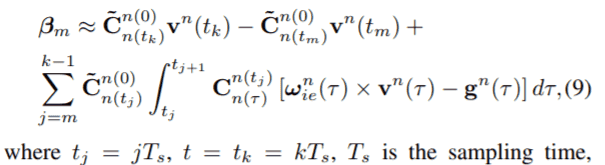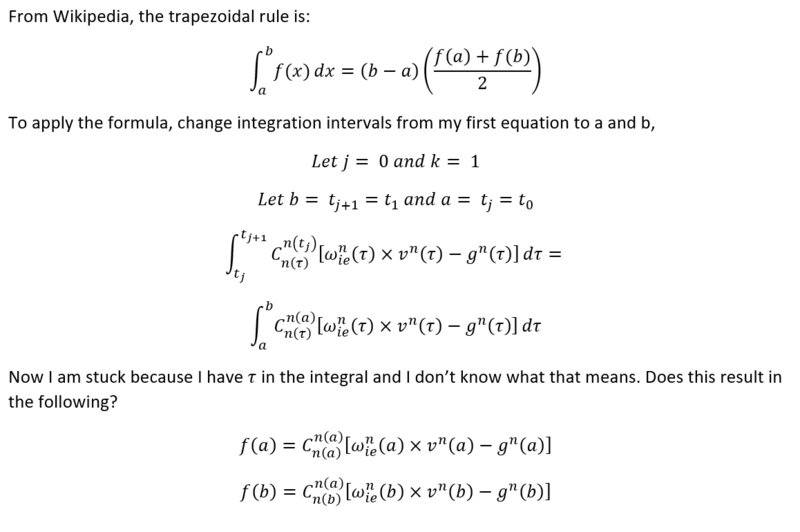# Discrete integration

• I
• 9988776655

#### 9988776655I have values for the variables (C, v, g, w at all sample points) but I do not know how to evaluate the integral. This equation is supposed to be implemented on a computer as part of a larger algorithm for navigation purposes. I have a feeling that the gyroscope sensor reading and or the accelerometer reading may be required to evaluate the integral but I am uncertain.

C is a 3x3 rotation matrix from subscript frame to superscript frame. Subscript and superscript n means the east north up frame.
m is the discrete point in time at the start of the data and k is the current discrete point in time.
v is a 1x3 vector of velocity, g is a 1x3 vector of gravity, w_ie_n is the 1x3 vector of Earth rate. X means the cross product. t refers to time.

The equation comes from the journal article:
A New Fast In-motion Coarse Alignment Method for GPS-aided Low-cost SINS (Yulong Huang, Yonggang Zhang)

How would I evaluate that integral?

#### Attachments

I think you have to do it numerically as you get new information in.

https://en.wikipedia.org/wiki/Numerical_integration

Programming-wise it depends on what language you choose and what level of library support it provides. MATLAB has code that can numerically integrate data provided. In Java, you'd have to write some code to evaluate each step of the integration (ie the stuff to the right of the integral sign over the range of the integral each time you get new data).

Two other languages to consider are Python with numpy, sympy and scipy support or Julia. Julia is hot right now and offers a coding experience similar to MATLAB but its free. Python with Conda is a popular implementation for Data Science projects.

I followed your link and I think that the trapezoidal rule will be useful. I am a MATLAB beginner so I will have to use Java. I am still confused about the integral though.